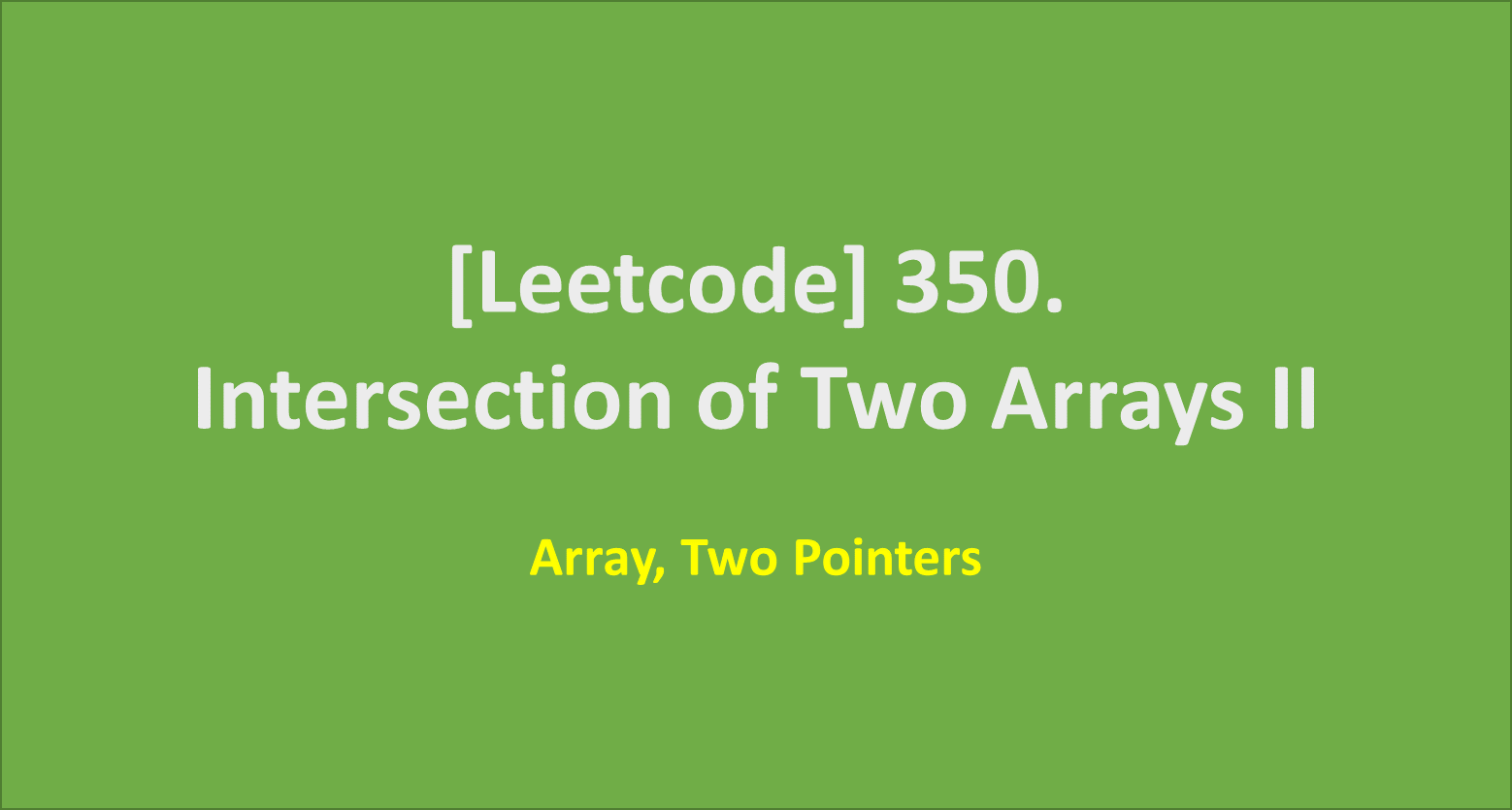# [Leetcode] 350. Intersection of Two Arrays II

limelimejiwon·2022년 3월 8일
0

## Python으로 공부하는 코딩테스트

목록 보기
6/67## 📄 Description

Given two integer arrays nums1 and nums2, return an array of their intersection. Each element in the result must appear as many times as it shows in both arrays and you may return the result in any order.

### Example 1:

Input: nums1 = [1,2,2,1], nums2 = [2,2]
Output: [2,2]

### Example 2:

Input: nums1 = [4,9,5], nums2 = [9,4,9,8,4]
Output: [4,9]
Explanation: [9,4] is also accepted.

## 🔨 My Solution

• sort the arrays
• use Two Pointers for pointing the each element of the arrays for comparision

#### Without Sorting the Arrays, Time Complexity will be worse!

Let's solve the problem without sorting the arrays. How will it work?
You have to compare every each of the elements in nums1 & nums2 array. So the Time Complexity will be O(n^2). Because there is no pattern or order in the arrays, so there is no other way to decrease the number of comparison.

But, If you sort both nums1 and nums2 arrays, you don't have to compare each elements in nums1 and nums2.

Let's see how it works.

Input will be like below.

nums1 = [4,9,5], nums2 = [9,4,9,8,4]

If you sort both arrays,

nums1 = [4,5,9], nums2 = [4,4,8,9,9]

nums1 = [4,5,9], nums2 = [4,4,8,9,9]
↑                ↑

It's same number, so both pointer will be moved to next.

nums1 = [4,5,9], nums2 = [4,4,8,9,9]
↑                ↑

It's not same, and number '5' is bigger than '4'.
Because both arrays are sorted in non-decreasing order, there is no doubt that
(1) there will not be same number as '4' in nums1 array.
(2) there might be same number as '5' in nums2 array.

Why? because nums1 array is sorted in non-decreasing order. So,
(1) move pointer for nums2 to the next.
(2) leave pointer for nums1 at current index.

Repeat the Steps above until one of the pointers gets to the end of the array, and you will get the Intersection of Two Arrays.

## 💻 My Submission

class Solution:
def intersect(self, nums1: List[int], nums2: List[int]) -> List[int]:
# set pointer for nums1(cur1) and nums2(cur2)
cur1 = cur2 = 0
# sort nums1 and nums2
nums1.sort()
nums2.sort()

# Repeat until one of the pointers gets to the end
while cur1!=len(nums1) and cur2!=len(nums2):
if nums1[cur1]==nums2[cur2]:
cur1+=1
cur2+=1
else:
if nums1[cur1]>nums2[cur2]:
cur2+=1
else:
cur1+=1
return answer

### 🔎 Complexity

Time Complexity will be affected by Sorting process & max(number of the two arrays).

Time Complexity: O(nlogn)+O(mlogm)+O(n) or O(m)
Space Complexity: O(n+m)